• Slides: 14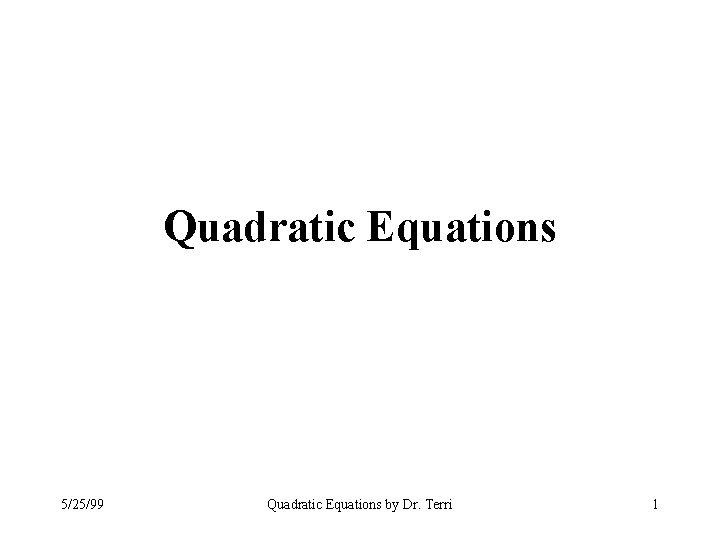Quadratic Equations A quadratic equation is of the form axn + bx + c = 0, where a, b, and c are real numbers and n > 1. Quadratic equations can be solved by • Factoring • Completing the square • Using the quadratic formula 5/25/99 Quadratic Equations by Dr. Terri 2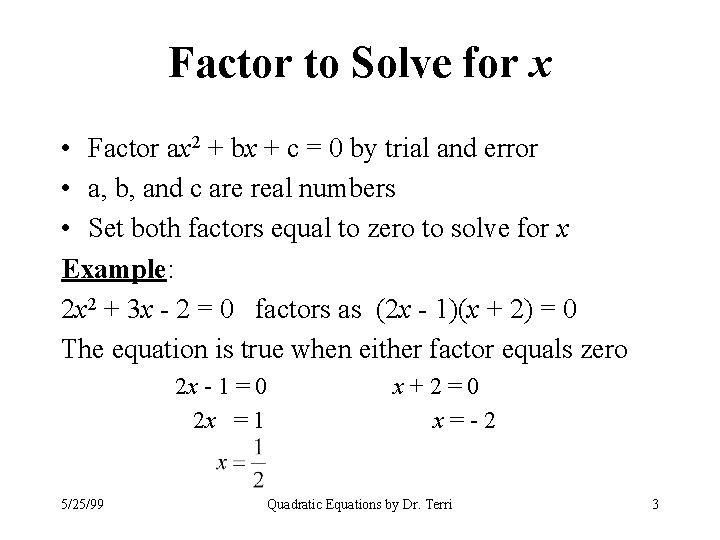Factor to Solve for x • Factor ax 2 + bx + c = 0 by trial and error • a, b, and c are real numbers • Set both factors equal to zero to solve for x Example: 2 x 2 + 3 x - 2 = 0 factors as (2 x - 1)(x + 2) = 0 The equation is true when either factor equals zero 2 x - 1 = 0 2 x = 1 5/25/99 x+2=0 x=-2 Quadratic Equations by Dr. Terri 3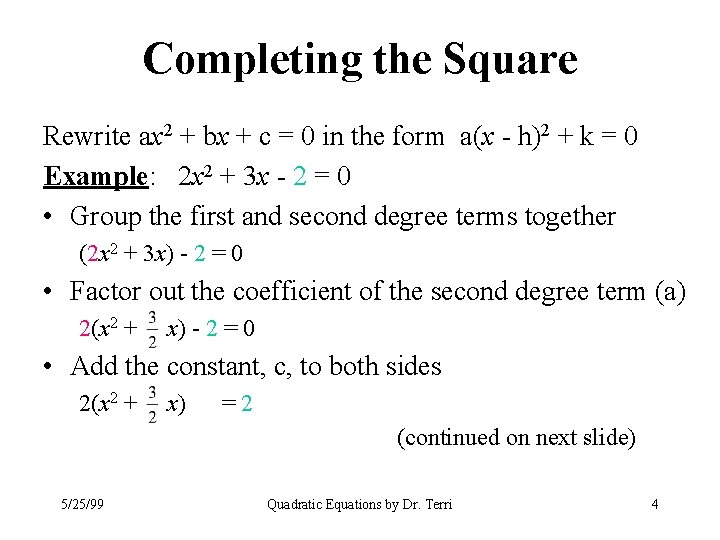Completing the Square Rewrite ax 2 + bx + c = 0 in the form a(x - h)2 + k = 0 Example: 2 x 2 + 3 x - 2 = 0 • Group the first and second degree terms together (2 x 2 + 3 x) - 2 = 0 • Factor out the coefficient of the second degree term (a) 2(x 2 + x) - 2 = 0 • Add the constant, c, to both sides 2(x 2 + x) =2 (continued on next slide) 5/25/99 Quadratic Equations by Dr. Terri 4Completing the Square 2(x 2 page 2 3 2 + x) = 2 • Take half the resulting coefficient of the first degree 3 term ( 2 ) ( 12 ) · ( 32 ) = 34 • Square the result 9 ( 34 )2 = 16 • Add in and subtract back out this new amount inside the parentheses 9 9 3 2 2(x + 2 x + 16 - 16 ) = 2 (continued on next slide) 5/25/99 Quadratic Equations by Dr. Terri 5Completing the Square page 3 • Determine the value of the last term inside the parentheses 2(- 9 ) = - 18 (multiply by the outside factor) 16 16 • Remove this last term from inside the parentheses 2(x 2 + 32 x + 169 ) - 18 =2 16 • Bring this value to the other side of the equation and reduce 25 2(x 2 + 32 x + 169 ) = 2 + 18 = 8 16 If you have trouble with fractions or reducing, review those lessons. (continued on next slide) 5/25/99 Quadratic Equations by Dr. Terri 6Completing the Square 2(x 2 3 2 9 16 page 4 25 =8 + x+ ) • Express the quantity inside the parentheses as the square of a binomial 2(x + 34 )2 = 25 (trial and error factoring) 8 • Divide both sides of the equation by the coefficient of the squared term 2 3 2 25. 1 25 (x + ) = = 2 4 8 2 16 • Take the square root of both sides of the equation x + 34 = 5 4 5/25/99 (continued on next slide) Quadratic Equations by Dr. Terri 7Completing the Square x+ 3 4 = 5 4 • Solve for x x + 34 = 54 and x 5/25/99 page 5 = 2 4 = 1 2 x+ x 3 4 = - 54 = - 84 = -2 Quadratic Equations by Dr. Terri 8Quadratic Formula to Solve for x The equation ax 2 + bx + c = 0 can be solved by substituting values for a, b, and c into the formula x = -b b 2 - 4 ac 2 a This formula is known as the quadratic formula. The quantity under the radical sign is known as the discriminant, and helps determine the nature of the resulting values for x. 5/25/99 Quadratic Equations by Dr. Terri 9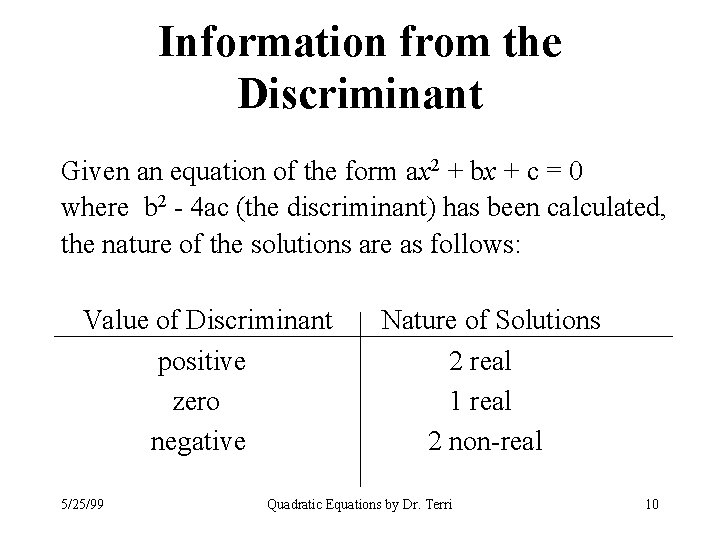Information from the Discriminant Given an equation of the form ax 2 + bx + c = 0 where b 2 - 4 ac (the discriminant) has been calculated, the nature of the solutions are as follows: Value of Discriminant positive zero negative 5/25/99 Nature of Solutions 2 real 1 real 2 non-real Quadratic Equations by Dr. Terri 10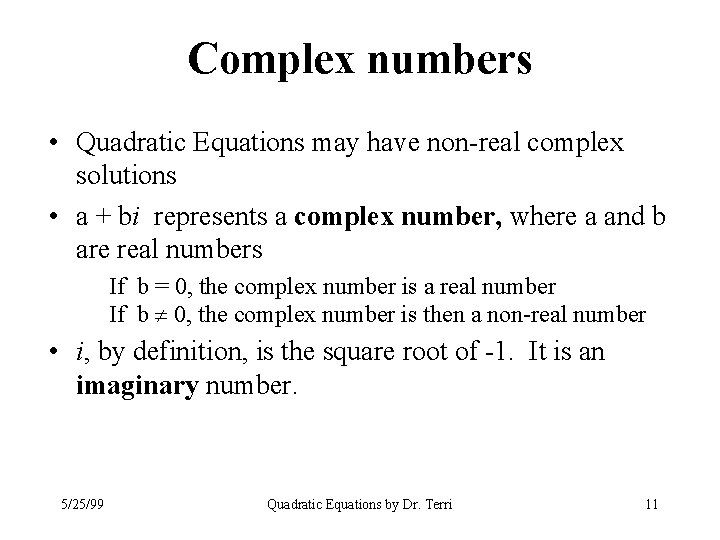Complex numbers • Quadratic Equations may have non-real complex solutions • a + bi represents a complex number, where a and b are real numbers If b = 0, the complex number is a real number If b 0, the complex number is then a non-real number • i, by definition, is the square root of -1. It is an imaginary number. 5/25/99 Quadratic Equations by Dr. Terri 11Complex Numbers page 2 Example: x 2 - 6 x + 13 = 0 This equation is not easily factored. It can be solved by completing the square or by using the quadratic formula. By using the quadratic formula, the solutions are: a = 1, b = -6, c = 13 5/25/99 Quadratic Equations by Dr. Terri 12Solving Quadratics: Exercises Solve -4 x 2 + x + 14 = 0 by factoring ( 4 x + 7) (-x + 2) = 0 answers: x = , x = 2 Solve 5/25/99 4 x 2 - 4 x + 1 = 9 by completing the square 4(x 2 - x + ) = 9 (lots of steps!) (x - )2 = x=+ x = 2 and x = -1 Quadratic Equations by Dr. Terri 13Solving Quadratics: Exercises Solve 7 x 2 + 5 = 0 x = -b b 2 - 4 ac 2 a x = -0 02 - 4. 7. 5 page 2 by the quadratic formula a = 7, b = 0, c = 5 14 x = -140 14 x = 2 i 35 = + i 35 14 7 5/25/99 Quadratic Equations by Dr. Terri 14